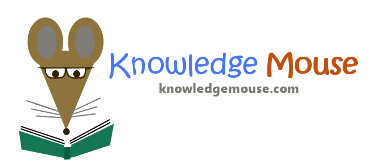1.
Where were you born?
A. 1 B. 1
2.
A. 1 B. 1
3.
Draw a smiley face with fangs.
A. 1 B. 1
4.
What is the capital of Kansas?
A. 1 B. 1
5.
Skip questions 1 to 4
A. 1 B. 1
6.
Write your name in the left corner
A. 1 B. 1
7.
1
A. 1 B. 1
8.
1
A. 1 B. 1

1.
Where were you born?
A. 1 B. 1
2.
A. 1 B. 1
3.
Draw a smiley face with fangs.
A. 1 B. 1
4.
What is the capital of Kansas?
A. 1 B. 1
5.
Skip questions 1 to 4
A. 1 B. 1
6.
Write your name in the left corner
A. 1 B. 1
7.
1
A. 1 B. 1
8.
1
A. 1 B. 1

#### Quick Feedback for Knowledge Mouse

Want to suggest a feature? Report a problem? Suggest a correction? Please let Knowledge Mouse know below: